# Testing GPT-3's Mathematics Comprehension

For the previous installment of this series with DALL-E 2, see here. I wanted to try GPT-3 on some similar problems. DALL-E clearly doesn't have a coherent world-model of the mathematical objects I'm asking it about; does GPT-3?

Plenty of people have already tested it on arithmetic so I won't waste much space on these, but here are a few I tried. (GPT-3's answers in green.)

• What is 45 times 29? 1,305 (Correct)
• What is 184 times 592? 109,568 (Should be 108,928)
• What is 2958 times 3395? 10,089,210 (Should be 10,042,410)
• What is 845,128,940 times 190,932,222? 1,614,853,339,040,188 (Should be 161,362,346,390,704,680)
• What is seven minus the square root of 25? 4 (Incorrect, should be 2.)
• What is the square root of 2? 1.41 (Correct first 3 digits (1.414...))
• What is the square root of 784? 28 (Correct)
• What is the square root of 15,376? 124 (Correct)
• What is the square root of 72,046,144?268 (Should be 8,488)
• What is the square root of 8,947?94.7 (Should be 94.588...)
• What is 1/7?0.142857142857.... (Correct)
• What is 298/1930?0.1547... (Should be 0.15440...)

With low numbers, it can usually get the exact answer. With larger numbers, it tends to get the right first few digits, but then messes it up. With irrational numbers it gives a few digits correctly and then gives up. With rational decimals it can get common ones correct and has similar problems with uncommon ones.

I'm more interested in geometric or topological problems, or other forms of abtract reasoning that a calculator can't perform.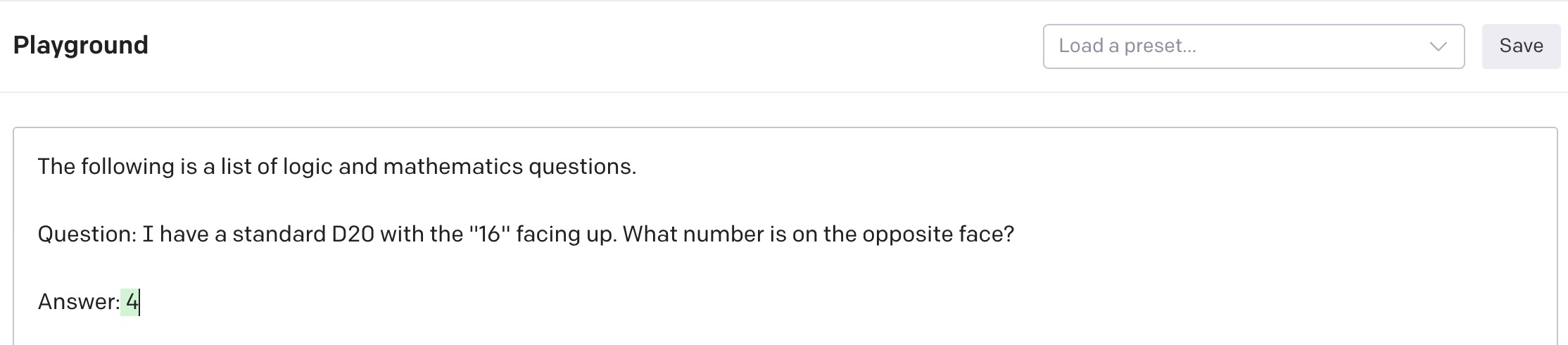Should be a 5; the convention is that opposing sides on a die always add up to 1 more than the highest face. This isn't really a math question though; it might just not know much about dice.

(For all the next questions I left the previous prompt in with a corrected answer, in case this helps it understand it needs to answer correctly.)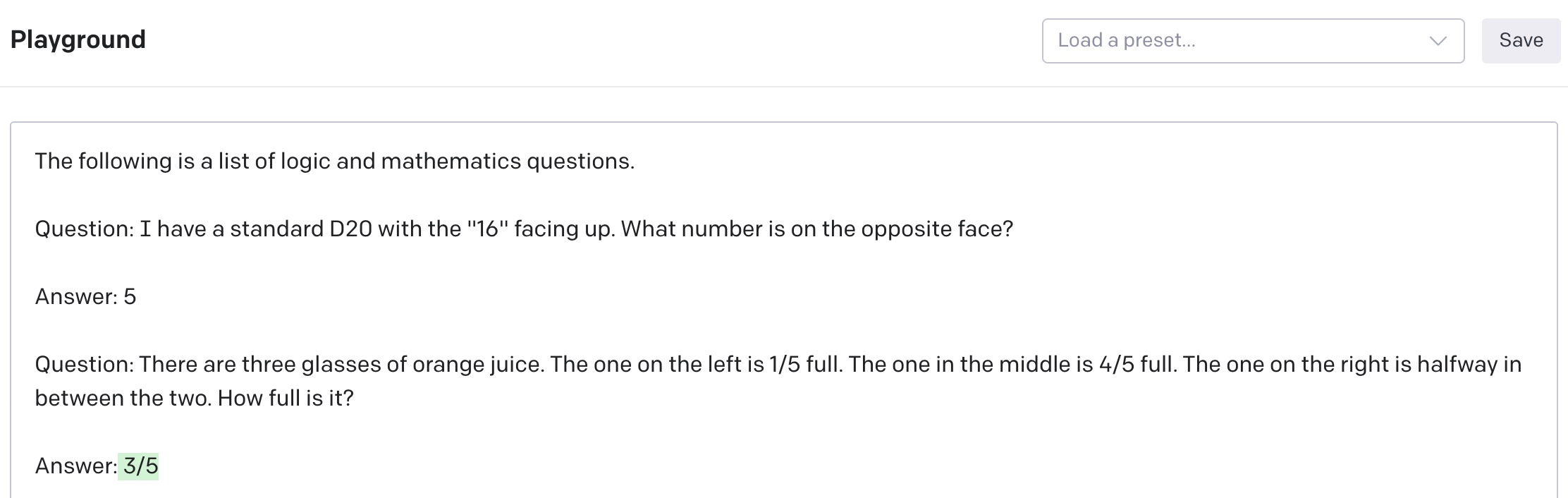Nope, doesn't understand fractions either. How about topology?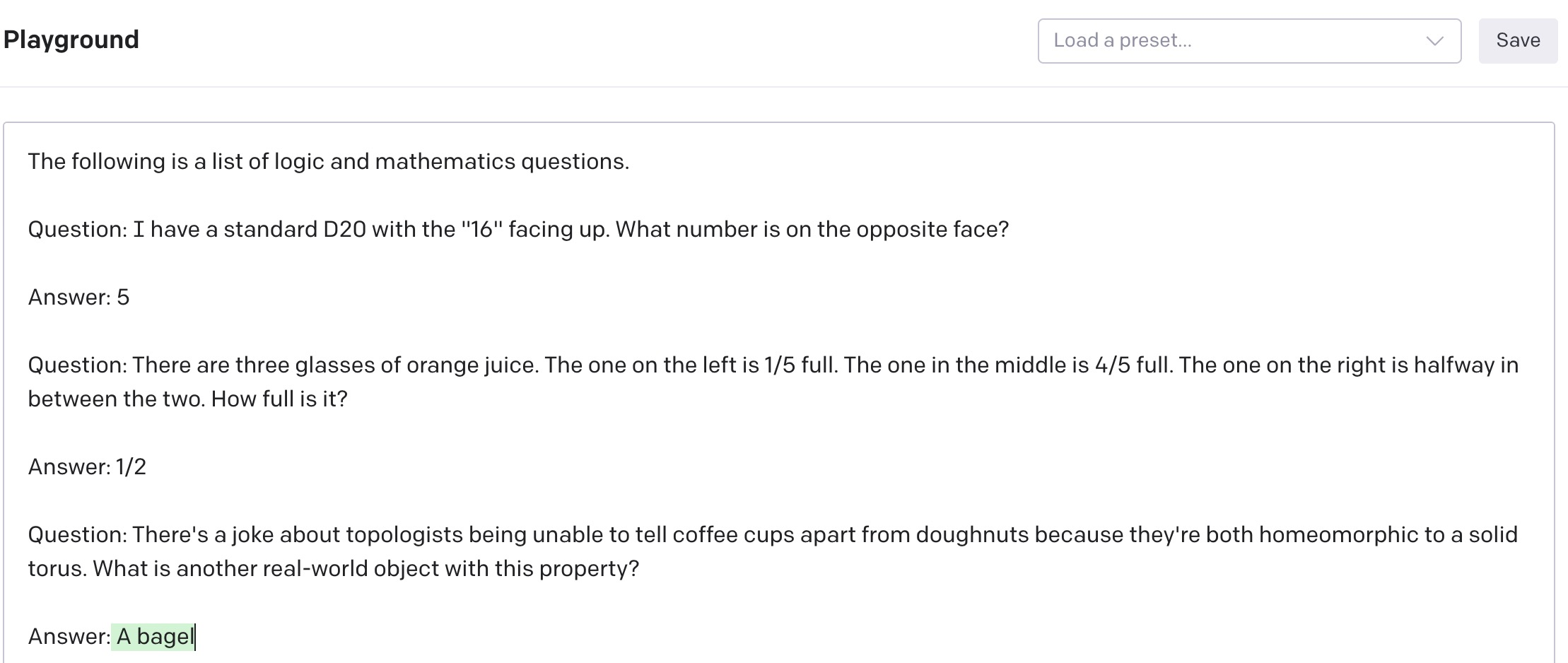I think this might just be because it recognizes that a bagel is extremely similar to a doughnut?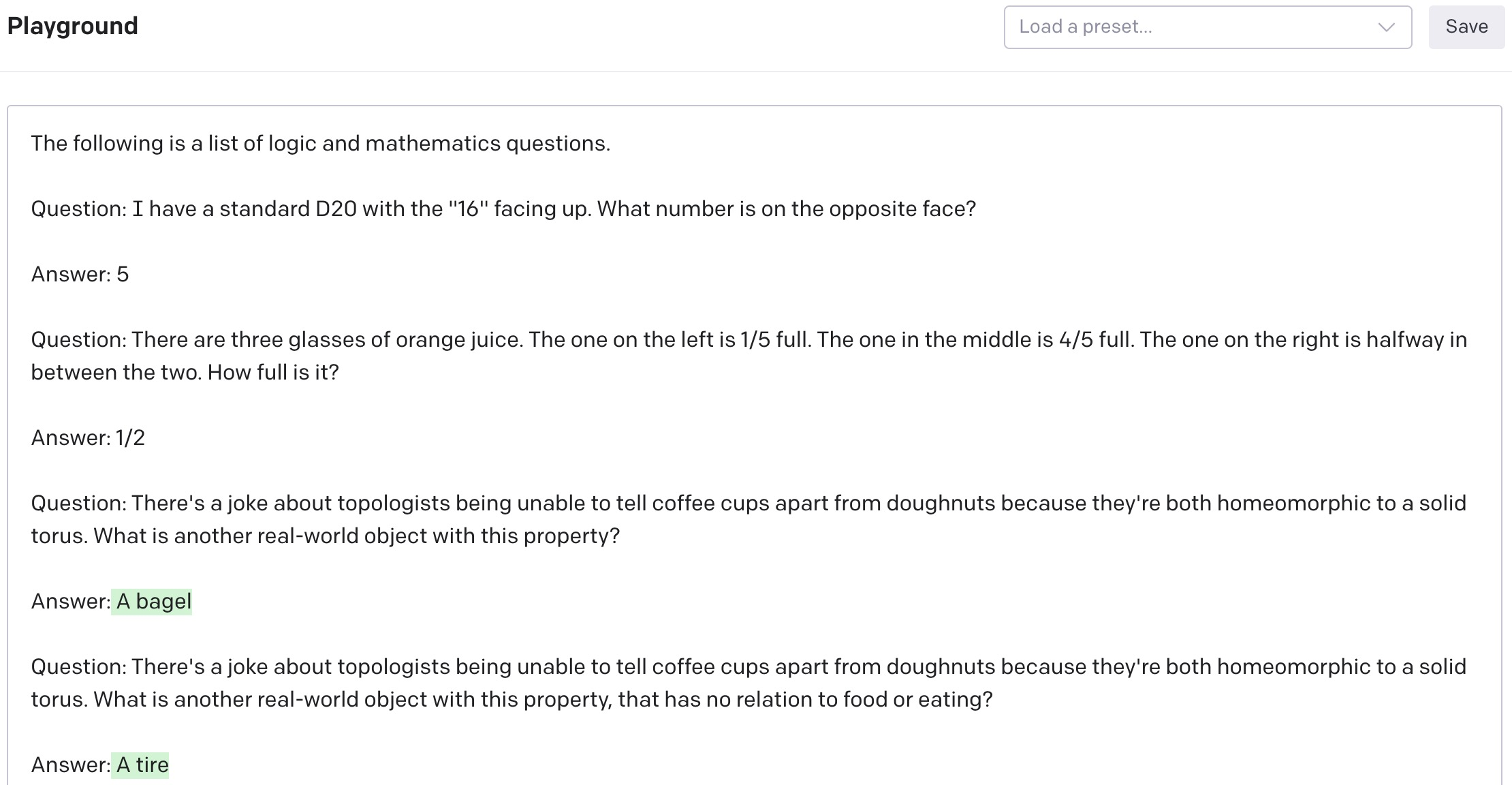Well that's scary. It... might know what a torus is.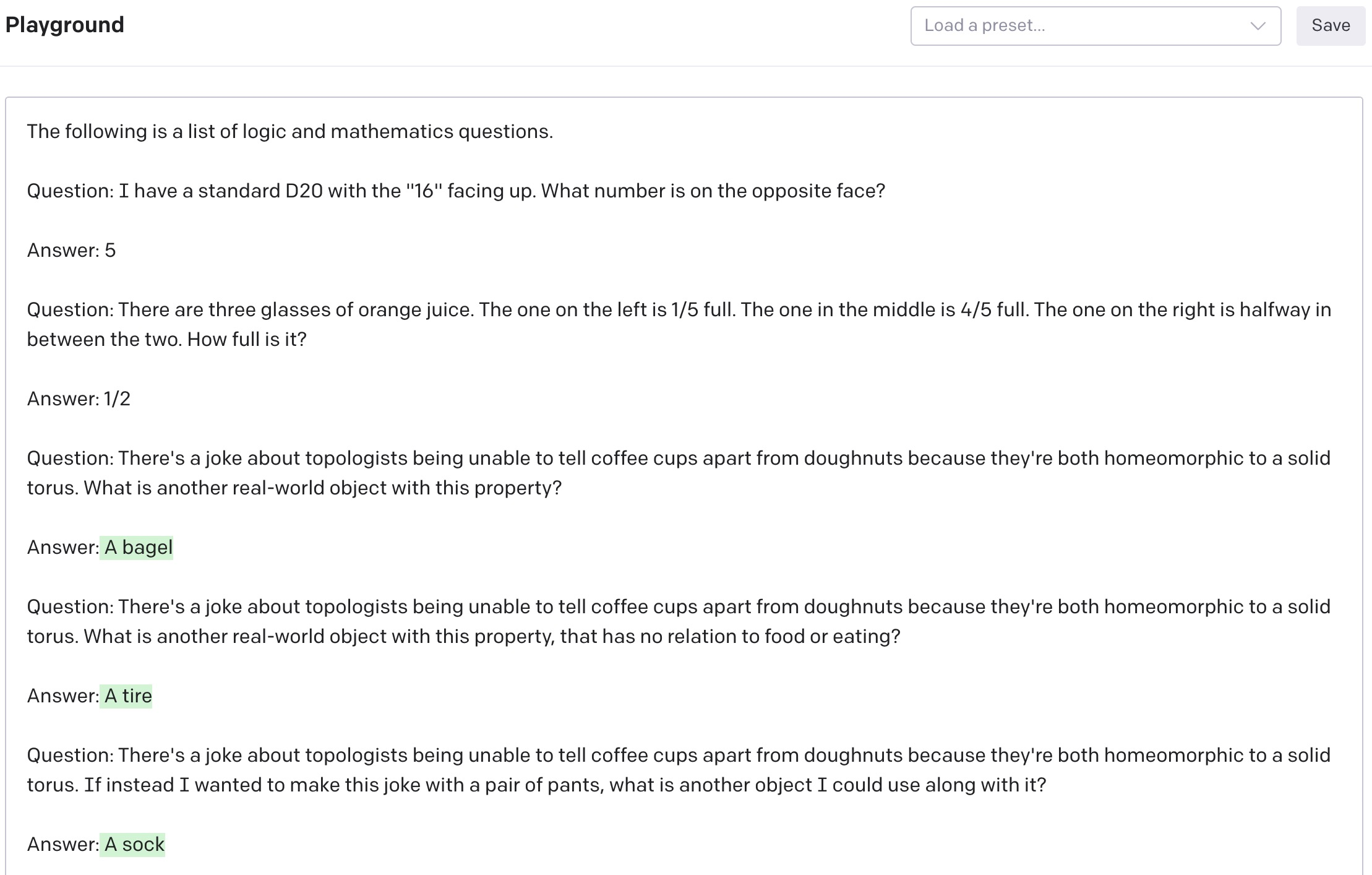Ok, we're not all doing to turn into paperclips quite yet. I'm really curious how it figured out the tire though.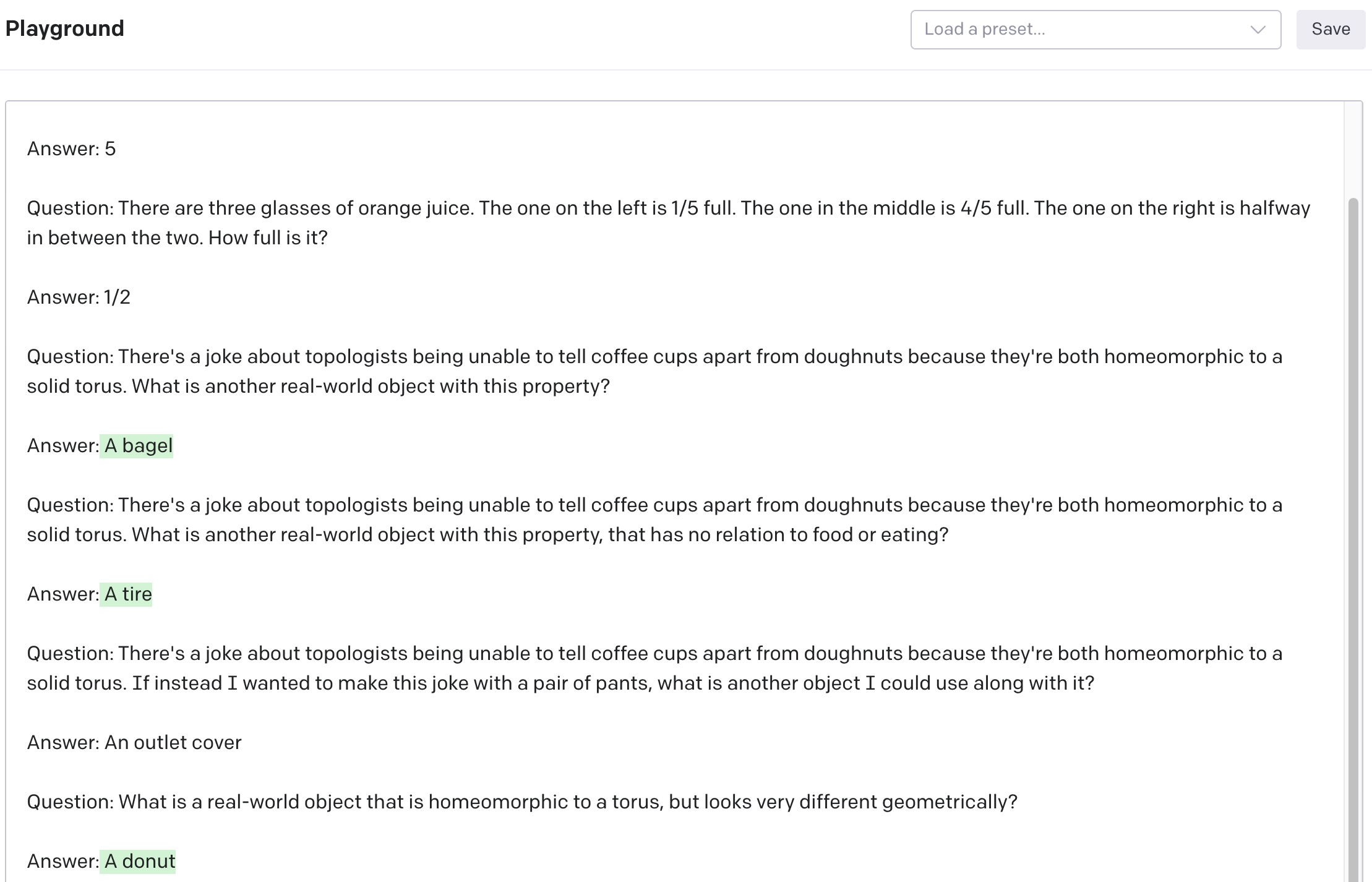Uh, no, that looks pretty similar.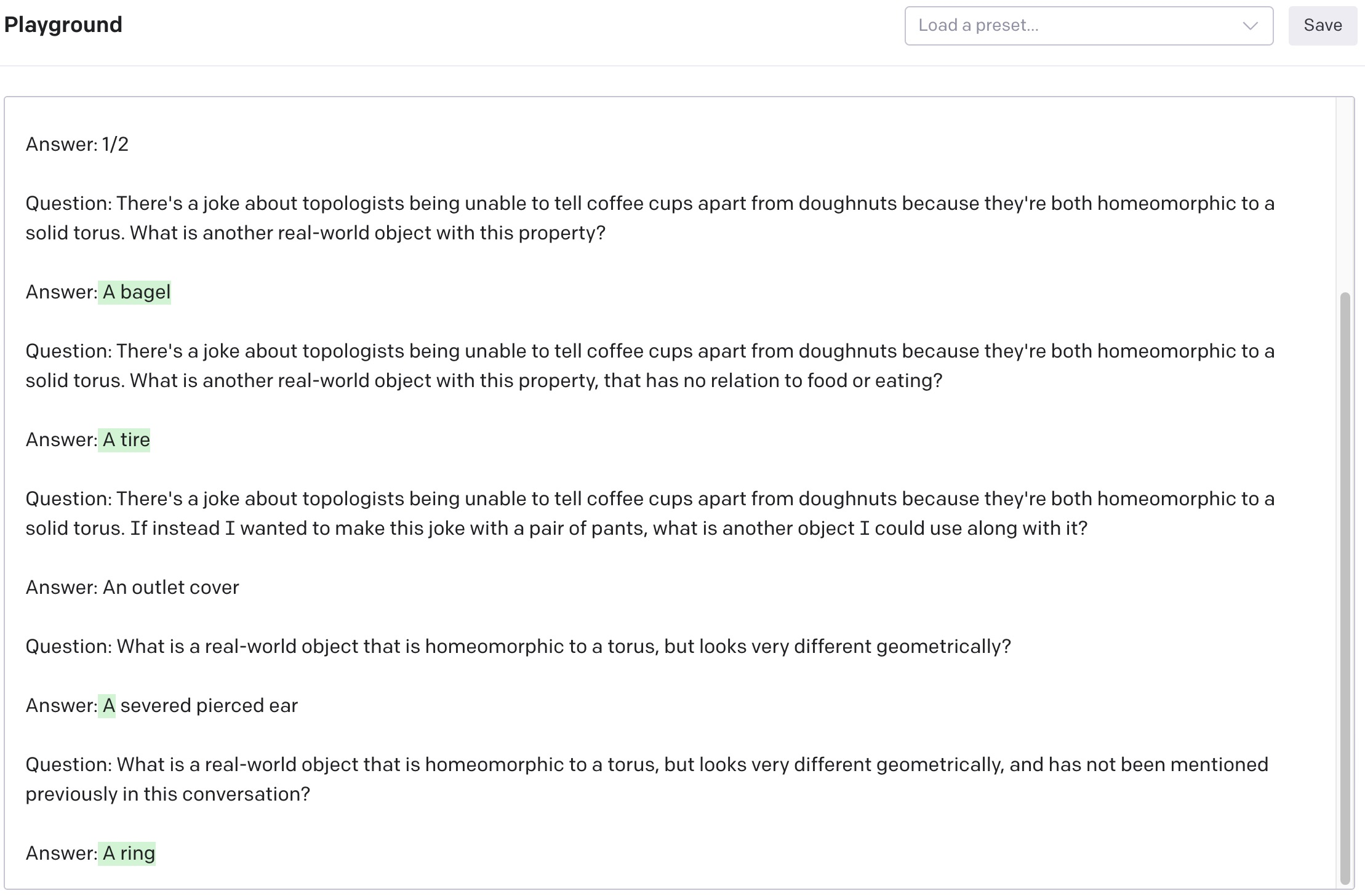A ring is still pretty toroidal geometrically, but that's somewhat subjective. I tried this prompt several times and got:

• A donut-shaped pillow
• A toilet paper roll
• A donut-shaped swimming pool
• A bagel
• A tennis ball
• A jellyfish
• A donut-shaped sponge
• A key ring
• A garden hose

Talking about a donut-shaped thing-that-is-very-different-from-a-donut doesn't quite satisfy what I asked for, but is a pretty clever hack.

Several of the other answers are wildly wrong, but the toilet paper roll and garden hose are correct.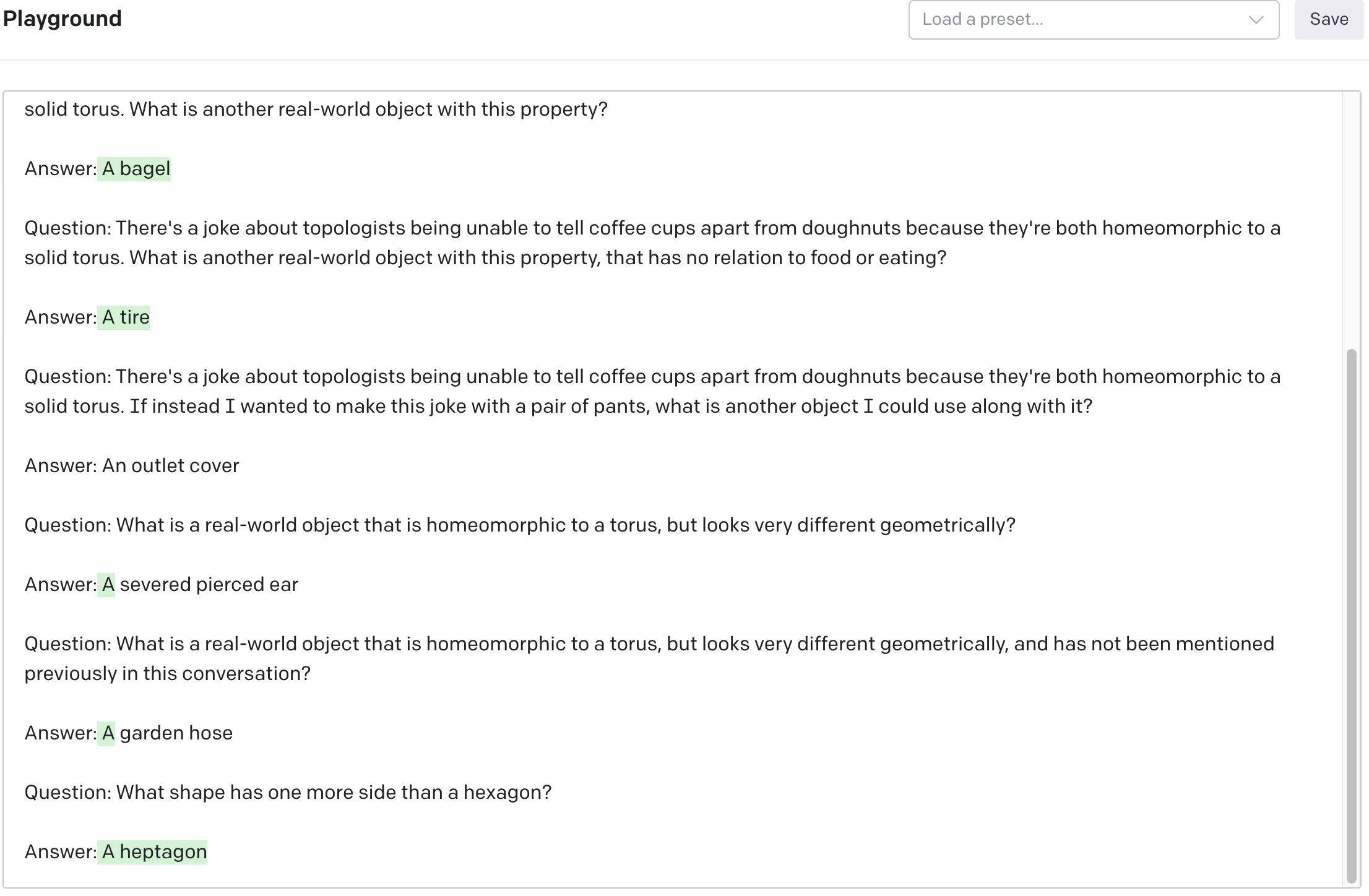Pretty good.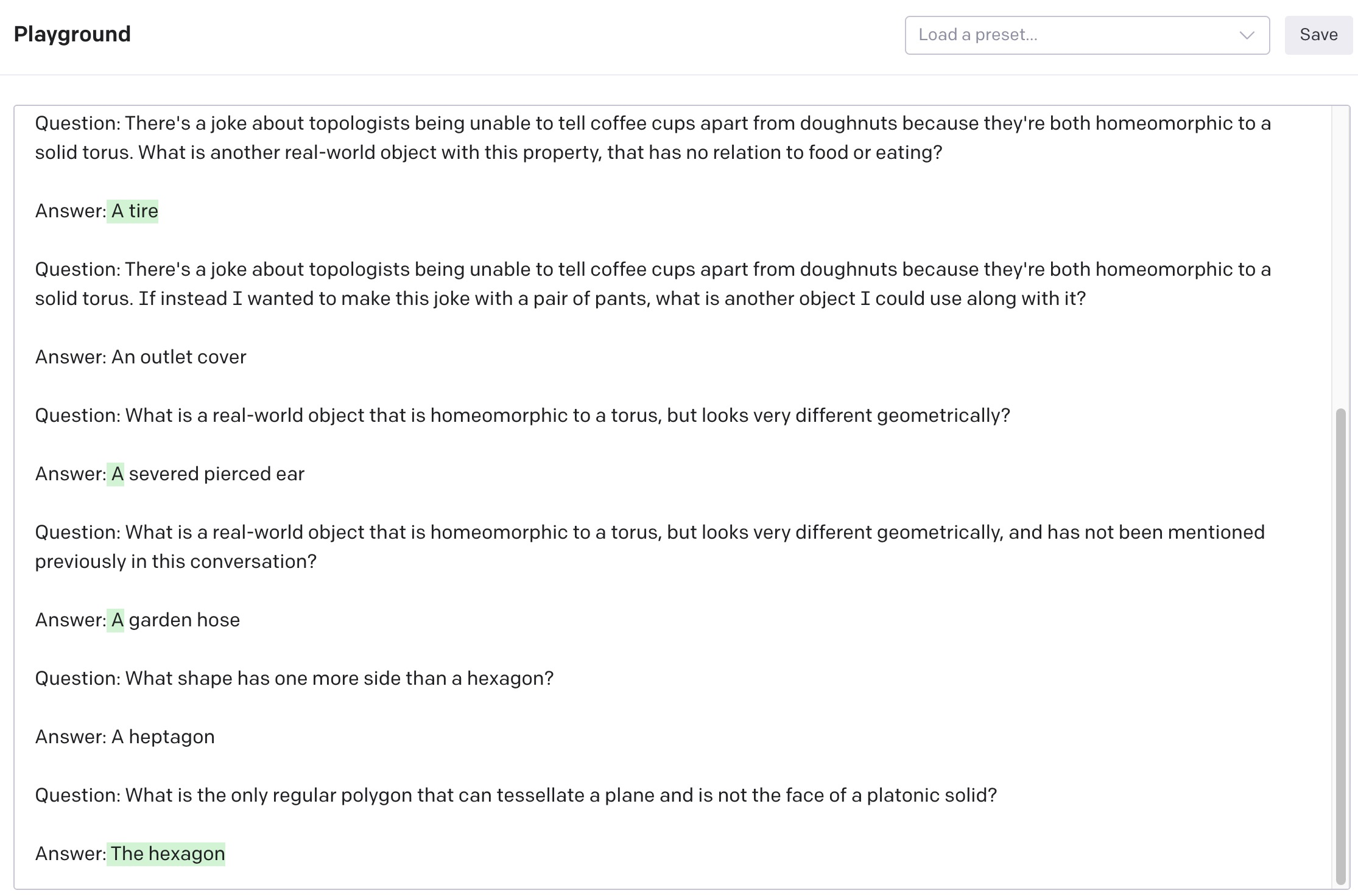Huh. Most humans could not answer that. That's seriously impressive. I tried this prompt several times to check it if got lucky, but no, it consistently answered either "The hexagon" or "A hexagon" every time.

GPT-3 is still pretty inconsistent. It does well on some problems and extremely poorly on others, seemingly arbitrarily. But its ability to consistently answer some pretty abstract questions makes me think it might actually have some primitive world models somewhere in there.